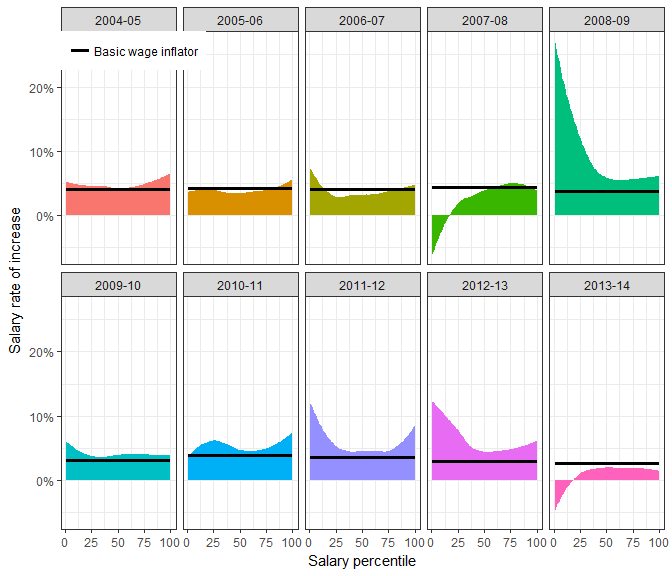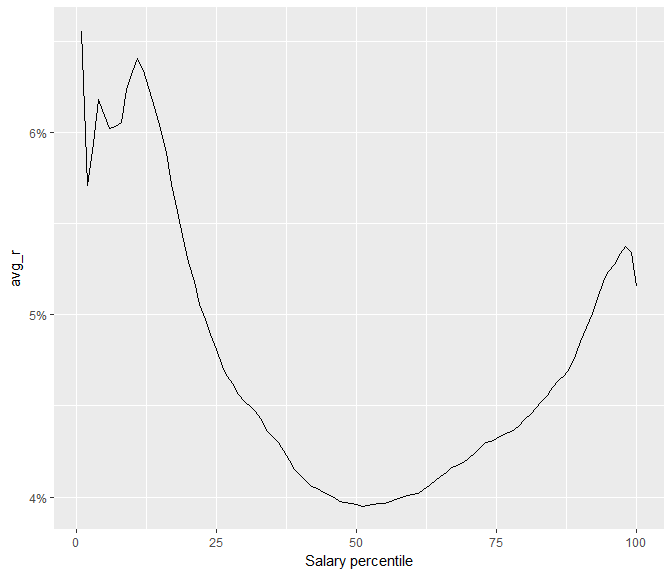# Differential uprating: more precise projections of population income

#### 2017-08-30

Since the most recent versions of data detailing population income and wealth (as is contained within the ATO’s sample files) is typically years out-of-date on release, even understanding the present requires a forecast. Since most variables relating to income increase due to inflation – so any sensible forecast will increase the values relative to the past – this procedure is often called uprating.

## Basic uprating

The most basic uprate method is the identity function, where the variables are not changed.

Alternatively, the variables can be increased by a long-term inflation rate. Even better, we can use the relevant time series indices for the variables and inflate using that index. For instance, salary and wage variables can be increased by the observed increase in the wage price index amd growth in the population of taxpayers by the labour force index.

## Differential uprating

Basic uprating suffers some limitations. There are significant structural differences in how much individuals’ incomes grow. High-income individuals tend to have higher than average wage growth. Low-income individuals also have higher than average wage growth. That is, the pattern of wage growth by wage is U-shaped.Differential uprating is a method by which variables are uprated by a function of not only the time period and the type of variable, but of the variable’s value too. To differentially uprate salary, use differentially_uprate_wage:

data_frame(wage = c(20e3, 50e3, 100e3)) %>%
mutate(ordinary = wage_inflator(wage, from_fy = "2012-13", to_fy = "2013-14"),
change ordinary = ordinary / wage - 1,
differential = differentially_uprate_wage(wage, from_fy = "2012-13", to_fy = "2013-14"),
change differential = differential / wage - 1
) %>%
mutate(wage = dollar(wage),
ordinary = dollar(ordinary),
differential = dollar(differential),
change ordinary = percent(change ordinary),
change differential = percent(change differential)) %>%
kable(align = rep("r", ncol(.)))
wage ordinary change ordinary differential change differential
$20,000$20,519 2.59% $20,580 2.900%$50,000 $51,296 2.59%$51,088 2.175%
$100,000$102,593 2.59% \$102,634 2.634%

Note that differentially_update_wage uses the 2003-04 to 2013-14 sample files to generate estimates of the shape of the U. So applying the function to any other vector may have surprising results. In particular, the distribution of the vector provided is not considered. Furthermore, the from_fy must be in the range 2003-04 to 2013-14.